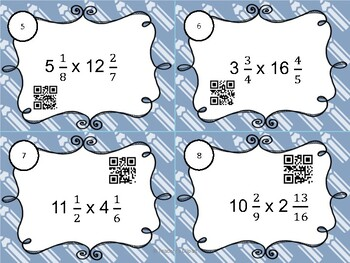Subject
Resource Type
File Type

PDF

(2 MB|17 pages)
Product Rating
Standards
• Product Description
• StandardsNEW

Do your students need practice multiplying fractions by fractions (whole numbers and order of operations)? This set of 24 task cards should will provide the practice they need. The cards start with basic questions and work up to more advanced questions (both number and word problems). Perfect for CC standard 5.NF.B and 6.NS.1

How can I use this product?

This set of multiplying fraction task cards can be used as a self-guided math center (QR codes are included for self checking), extra practice for early finishers or small group tutoring, or scoot around the room.

What is included?

1 pdf file with 17 pages

* 2 pages of cover/copyright info

* 6 pages (with four on a page) without QR Codes

* 6 pages (with four on a page) with QR codes

* 2 recording sheet options

Interpret and compute quotients of fractions, and solve word problems involving division of fractions by fractions, e.g., by using visual fraction models and equations to represent the problem. For example, create a story context for (2/3) ÷ (3/4) and use a visual fraction model to show the quotient; use the relationship between multiplication and division to explain that (2/3) ÷ (3/4) = 8/9 because 3/4 of 8/9 is 2/3. (In general, (𝘢/𝘣) ÷ (𝘤/𝘥) = 𝘢𝘥/𝘣𝘤.) How much chocolate will each person get if 3 people share 1/2 lb of chocolate equally? How many 3/4-cup servings are in 2/3 of a cup of yogurt? How wide is a rectangular strip of land with length 3/4 mi and area 1/2 square mi?
Interpret a fraction as division of the numerator by the denominator (𝘢/𝘣 = 𝘢 ÷ 𝘣). Solve word problems involving division of whole numbers leading to answers in the form of fractions or mixed numbers, e.g., by using visual fraction models or equations to represent the problem. For example, interpret 3/4 as the result of dividing 3 by 4, noting that 3/4 multiplied by 4 equals 3, and that when 3 wholes are shared equally among 4 people each person has a share of size 3/4. If 9 people want to share a 50-pound sack of rice equally by weight, how many pounds of rice should each person get? Between what two whole numbers does your answer lie?
Total Pages
17 pages
Included
Teaching Duration
N/A
Report this Resource to TpT
Reported resources will be reviewed by our team. Report this resource to let us know if this resource violates TpT’s content guidelines.